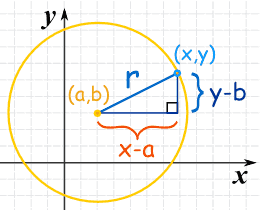# Equation of Circle

The equation of circle is:$\boxed{(x-a)^2+(y-b)^2=r^2}$

Centre: (a,b)

## Memory Tip

• The equation is actually Pythagoras’ Theorem !!!
• Please take note of the minus sign “(x-a), (y-b)”## Author: mathtuition88

https://mathtuition88.com/

This site uses Akismet to reduce spam. Learn how your comment data is processed.